# Running a model on separate groups

Ever wanted to run a model on separate groups of data? Read on!

Here’s an example of a regression model fitted to separate groups: predicting a car’s Miles per Gallon with various attributes, but spearately for automatic and manual cars.

``````library(tidyverse)
library(broom)
mtcars %>%
nest(-am) %>%
mutate(am = factor(am, levels = c(0, 1), labels = c("automatic", "manual")),
fit = map(data, ~ lm(mpg ~ hp + wt + disp, data = .)),
results = map(fit, augment)) %>%
unnest(results) %>%
ggplot(aes(x = mpg, y = .fitted)) +
geom_abline(intercept = 0, slope = 1, alpha = .2) +  # Line of perfect fit
geom_point() +
facet_grid(am ~ .) +
labs(x = "Miles Per Gallon", y = "Predicted Value") +
theme_bw()
``````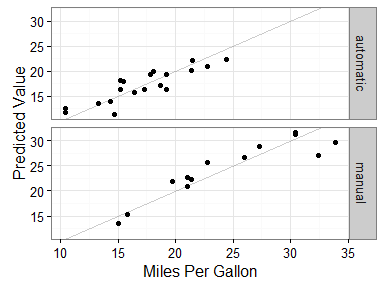## Getting Started #

A few things to do/keep in mind before getting started…

### A lot of detail for novices #

I started this post after working on a larger problem for which I couldn’t add detail about lower-level aspects. So this post is very detailed about a particular aspect of a larger problem and, thus, best suited for novice to intermediate R users.

### One of many approaches #

There are many ways to tackle this problem. We’ll cover a particular approach that I like, but be mindful that there are plenty of alternatives out there.

### The Tidyverse #

We’ll be using functions from many tidyverse packages like dplyr and ggplot2, as well as the tidy modelling package broom. If you’re unfamiliar with these and want to learn more, a good place to get started is Hadley Wickham’s R for Data Science. Let’s load these as follows (making use of the new tidyverse package):

``````library(tidyverse)
library(broom)
``````

### mtcars #

Ah, `mtcars`. My favourite data set. We’re gong to use this data set for most examples. Be sure to check it out if you’re unfamiliar with it! Run `?mtcars`, or here’s a quick reminder:

``````head(mtcars)
#>                    mpg cyl disp  hp drat    wt  qsec vs am gear carb
#> Mazda RX4         21.0   6  160 110 3.90 2.620 16.46  0  1    4    4
#> Mazda RX4 Wag     21.0   6  160 110 3.90 2.875 17.02  0  1    4    4
#> Datsun 710        22.8   4  108  93 3.85 2.320 18.61  1  1    4    1
#> Hornet 4 Drive    21.4   6  258 110 3.08 3.215 19.44  1  0    3    1
#> Hornet Sportabout 18.7   8  360 175 3.15 3.440 17.02  0  0    3    2
#> Valiant           18.1   6  225 105 2.76 3.460 20.22  1  0    3    1
``````

Let’s get to it.

## Nesting Tibbles #

Nested tibbles - sounds like some rare bird! For those who aren’t familiar with them, “tibbles are a modern take on data frames”. For our purposes here, you can think of a tibble like a data frame. It just prints to the console a little differently. Click the quote to learn more from the tibble vignette.

So what do I mean by nested tibbles? Well, this is when we take sets of columns and rows from one data frame/tibble, and save (nest) them as cells in a new tibble. Make sense? No? Not to worry. An example will likley explain better.

We do this with `nest()` from the tidyr package (which is loaded with `library(tidyverse)`). Perhaps the most common use of this function, and exactly how we’ll use it, is to pipe in a tibble or data frame, and drop one or more categorical variables using `-`. For example, let’s `nest()` the `mtcars` data set and drop the cylinder (`cyl`) column:

``````mtcars %>% nest(-cyl)
#> # A tibble: 3 × 2
#>     cyl               data
#>   <dbl>             <list>
#> 1     6  <tibble [7 × 10]>
#> 2     4 <tibble [11 × 10]>
#> 3     8 <tibble [14 × 10]>
``````

This looks interesting. We have one column that makes sense: `cyl` lists each of the levels of the cylinder variable. But what’s that `data` colum? Looks like tibbles. Let’s look into the tibble in the row where `cyl == 4` to learn more:

``````d <- mtcars %>% nest(-cyl)
d\$data[d\$cyl == 4]
#> []
#> # A tibble: 11 × 10
#>      mpg  disp    hp  drat    wt  qsec    vs    am  gear  carb
#>    <dbl> <dbl> <dbl> <dbl> <dbl> <dbl> <dbl> <dbl> <dbl> <dbl>
#> 1   22.8 108.0    93  3.85 2.320 18.61     1     1     4     1
#> 2   24.4 146.7    62  3.69 3.190 20.00     1     0     4     2
#> 3   22.8 140.8    95  3.92 3.150 22.90     1     0     4     2
#> 4   32.4  78.7    66  4.08 2.200 19.47     1     1     4     1
#> 5   30.4  75.7    52  4.93 1.615 18.52     1     1     4     2
#> 6   33.9  71.1    65  4.22 1.835 19.90     1     1     4     1
#> 7   21.5 120.1    97  3.70 2.465 20.01     1     0     3     1
#> 8   27.3  79.0    66  4.08 1.935 18.90     1     1     4     1
#> 9   26.0 120.3    91  4.43 2.140 16.70     0     1     5     2
#> 10  30.4  95.1   113  3.77 1.513 16.90     1     1     5     2
#> 11  21.4 121.0   109  4.11 2.780 18.60     1     1     4     2
``````

This looks a bit like the `mtcars` data, but did you notice that the `cyl` column isn’t there and that there’s only 11 rows? This is because we see a subset of the complete `mtcars` data set where `cyl == 4`. By using `nest(-cyl)`, we’ve collapsed the entire `mtcars` data set into two columns and three rows (one for each category in `cyl`).

Aside, it’s easy to dissect data by multiple categorical variables further by dropping them in `nest()`. For example, we can nest our data by the number of cylinders AND whether the car is automatic or manual (`am`) as follows:

``````mtcars %>% nest(-cyl, -am)
#> # A tibble: 6 × 3
#>     cyl    am              data
#>   <dbl> <dbl>            <list>
#> 1     6     1  <tibble [3 × 9]>
#> 2     4     1  <tibble [8 × 9]>
#> 3     6     0  <tibble [4 × 9]>
#> 4     8     0 <tibble [12 × 9]>
#> 5     4     0  <tibble [3 × 9]>
#> 6     8     1  <tibble [2 × 9]>
``````

If you compare carefully to the above, you’ll notice that each tibble in `data` has 9 columns instead of 10. This is because we’ve now extracted `am`. Also, there are far fewer rows in each tibble. This is because each tibble contains a much smaller subset of the data. E.g., instead of all the data for cars with 4 cylinders being in one cell, this data is further split into two cells – one for automatic, and one for manual cars.

## Fitting models to nested data #

Now that we can separate data for each group(s), we can fit a model to each tibble in `data` using `map()` from the purrr package (also `tidyverse`). We’re going to add the results to our existing tibble using `mutate()` from the dplyr package (again, `tidyverse`). Here’s a generic version of our pipe with adjustable parts in caps:

``````DATA_SET %>%
nest(-CATEGORICAL_VARIABLE) %>%
mutate(fit = map(data, ~ MODEL_FUNCTION(...)))
``````

Where you see `...`, using a single dot (`.`) will represent each nested tibble

Let’s start with a silly but simple example: a student t-test examining whether `mpg` is significantly greater than 0 for each group of cars with different cylinders:

``````mtcars %>%
nest(-cyl) %>%
mutate(fit = map(data, ~ t.test(.\$mpg)))
#> # A tibble: 3 × 3
#>     cyl               data         fit
#>   <dbl>             <list>      <list>
#> 1     6  <tibble [7 × 10]> <S3: htest>
#> 2     4 <tibble [11 × 10]> <S3: htest>
#> 3     8 <tibble [14 × 10]> <S3: htest>
``````

We’ll talk about the new `fit` column in a moment. First, let’s discuss the new line, `mutate(fit = map(data, ~ t.test(.\$mpg)))`:

• `mutate(fit = ...)` is a dplyr function that will add a new column to our tibble called `fit`.
• `map(data, ...)` is a purrr function that iterates through each cell of the `data` column (which has our nested tibbles).
• `~ t.test(.\$mpg)` is running the t.test for each cell. Because this takes place within `map()`, we must start with `~`, and use `.` whenever we want to reference the nested tibble that is being iterated on.

What’s each `<S3: htest>` in the `fit` column? It’s the fitted `t.test()` model for each nested tibble. Just like we peeked into a single `data` cell, let’s look into a single `fit` cell - for cars with 4 cylinders:

``````d <- mtcars %>%
nest(-cyl) %>%
mutate(fit = map(data, ~ t.test(.\$mpg)))
d\$fit[d\$cyl == 4]
#> []
#>
#>  One Sample t-test
#>
#> data:  .\$mpg
#> t = 19.609, df = 10, p-value = 2.603e-09
#> alternative hypothesis: true mean is not equal to 0
#> 95 percent confidence interval:
#>  23.63389 29.69338
#> sample estimates:
#> mean of x
#>  26.66364
``````

Looking good. So we now know how to `nest()` a data set by one or more groups, and fit a statistical model to the data corresponding to each group.

## Extracting fit information #

Our final goal is to obtain useful information from the fitted models. We could manually look into each `fit` cell, but this is tedious. Instead, we’ll extract information from our fitted models by adding one or more lines to `mutate()`, and using `map_*(fit, ...)` to iterate through each fitted model. For example, the following extracts the `p.values` from each t.test into a new column called `p`:

``````mtcars %>%
nest(-cyl) %>%
mutate(fit = map(data, ~ t.test(.\$mpg)),
p   = map_dbl(fit, "p.value"))
#> # A tibble: 3 × 4
#>     cyl               data         fit            p
#>   <dbl>             <list>      <list>        <dbl>
#> 1     6  <tibble [7 × 10]> <S3: htest> 3.096529e-08
#> 2     4 <tibble [11 × 10]> <S3: htest> 2.602733e-09
#> 3     8 <tibble [14 × 10]> <S3: htest> 1.092804e-11
``````

`map_dbl()` is used because we want to return a number (a “double”) rather than a list of objects (which is what `map()` does). Explaining the variants of `map()` and how to use them is well beyond the scope of this post. The important point here is that we can iterate through our fitted models in the `fit` column to extract information for each group of data. For more details, I recommend reading the “The Map Functions” in R for Data Science.

### broom and unnest() #

In addition to extracting a single value like above, we can extract entire data frames of information generated via functions from the broom package (which are available for most of the common models in R). For example, the `glance()` function returns a one-row data frame of model information. Let’s extract this information into a new column called `results`:

``````mtcars %>%
nest(-cyl) %>%
mutate(fit = map(data, ~ t.test(.\$mpg)),
results = map(fit, glance))
#> # A tibble: 3 × 4
#>     cyl               data         fit              results
#>   <dbl>             <list>      <list>               <list>
#> 1     6  <tibble [7 × 10]> <S3: htest> <data.frame [1 × 8]>
#> 2     4 <tibble [11 × 10]> <S3: htest> <data.frame [1 × 8]>
#> 3     8 <tibble [14 × 10]> <S3: htest> <data.frame [1 × 8]>
``````

If you extract information like this, the next thing you’re likely to want to do is `unnest()` it as follows:

``````mtcars %>%
nest(-cyl) %>%
mutate(fit = map(data, ~ t.test(.\$mpg)),
results = map(fit, glance)) %>%
unnest(results)
#> # A tibble: 3 × 11
#>     cyl               data         fit estimate statistic      p.value
#>   <dbl>             <list>      <list>    <dbl>     <dbl>        <dbl>
#> 1     6  <tibble [7 × 10]> <S3: htest> 19.74286  35.93552 3.096529e-08
#> 2     4 <tibble [11 × 10]> <S3: htest> 26.66364  19.60901 2.602733e-09
#> 3     8 <tibble [14 × 10]> <S3: htest> 15.10000  22.06952 1.092804e-11
#> # ... with 5 more variables: parameter <dbl>, conf.low <dbl>,
#> #   conf.high <dbl>, method <fctr>, alternative <fctr>
``````

We’ve now unnested all of the model information, which includes the t value (`statistic`), the p value (`p.value`), and many others.

We can do whatever we want with this information. For example, the below plots the group `mpg` means with confidence intervals generated by the t.test:

``````mtcars %>%
nest(-cyl) %>%
mutate(fit = map(data, ~ t.test(.\$mpg)),
results = map(fit, glance)) %>%
unnest(results) %>%
ggplot(aes(x = factor(cyl), y = estimate)) +
geom_bar(stat = "identity") +
geom_errorbar(aes(ymin = conf.low, ymax = conf.high), width = .2) +
labs(x = "Cylinders (cyl)", y = "Miles Per Gallon (mpg)")
``````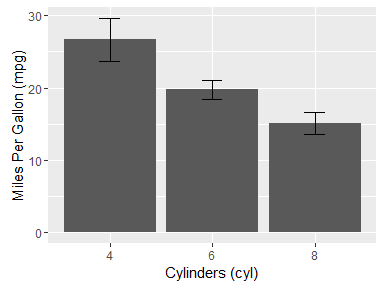## Regression #

Let’s push ourselves and see if we can do the same sort of thing for liner regression. Say we want to examine whether the prediction of `mpg` by `hp`, `wt` and `disp`, differs for cars with different numbers of cylinders. The first significant change will be our `fit` variable, created as follows:

``````mtcars %>%
nest(-cyl) %>%
mutate(fit = map(data, ~ lm(mpg ~ hp + wt + disp, data = .)))
#> # A tibble: 3 × 3
#>     cyl               data      fit
#>   <dbl>             <list>   <list>
#> 1     6  <tibble [7 × 10]> <S3: lm>
#> 2     4 <tibble [11 × 10]> <S3: lm>
#> 3     8 <tibble [14 × 10]> <S3: lm>
``````

That’s it! Notice how everything else is the same. All we’ve done is swapped out a `t.test()` for `lm()`, using our variables and data in the appropriate places. Let’s `glance()` at the model:

``````mtcars %>%
nest(-cyl) %>%
mutate(fit = map(data, ~ lm(mpg ~ hp + wt + disp, data = .)),
results = map(fit, glance)) %>%
unnest(results)
#> # A tibble: 3 × 14
#>     cyl               data      fit r.squared adj.r.squared    sigma
#>   <dbl>             <list>   <list>     <dbl>         <dbl>    <dbl>
#> 1     6  <tibble [7 × 10]> <S3: lm> 0.7217114     0.4434228 1.084421
#> 2     4 <tibble [11 × 10]> <S3: lm> 0.7080702     0.5829574 2.912394
#> 3     8 <tibble [14 × 10]> <S3: lm> 0.4970692     0.3461900 2.070017
#> # ... with 8 more variables: statistic <dbl>, p.value <dbl>, df <int>,
#> #   logLik <dbl>, AIC <dbl>, BIC <dbl>, deviance <dbl>, df.residual <int>
``````

We haven’t added anything we haven’t seen already. Let’s go and plot the R-squared values to see just how much variance is accounted for in each model:

``````mtcars %>%
nest(-cyl) %>%
mutate(fit = map(data, ~ lm(mpg ~ hp + wt + disp, data = .)),
results = map(fit, glance)) %>%
unnest(results) %>%
ggplot(aes(x = factor(cyl), y = r.squared)) +
geom_bar(stat = "identity") +
labs(x = "Cylinders", y = expression(R^{2}))
``````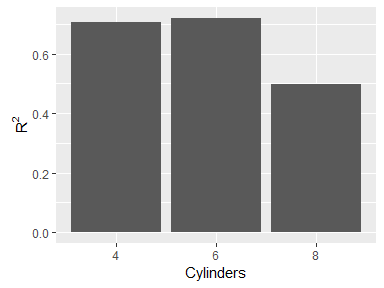It looks to me like the model performs poorer for cars with 8 cylinders than cars with 4 or 6 cylinders.

## Row-wise values and `augment()`#

We’ll cover one final addition: extracting row-wise data with broom’s `augment()` function. Unlike `glance()`, `augment()` extracts information that matches every row of the original data such as the predicted and residual values. If we have a model that `augment()` works with, we can add it to our mutate call just as we added `glance()`. Let’s swap out `glance()` for `augment()` in the regression model above:

``````mtcars %>%
nest(-cyl) %>%
mutate(fit = map(data, ~ lm(mpg ~ hp + wt + disp, data = .)),
results = map(fit, augment))
#> # A tibble: 3 × 4
#>     cyl               data      fit                results
#>   <dbl>             <list>   <list>                 <list>
#> 1     6  <tibble [7 × 10]> <S3: lm>  <data.frame [7 × 11]>
#> 2     4 <tibble [11 × 10]> <S3: lm> <data.frame [11 × 11]>
#> 3     8 <tibble [14 × 10]> <S3: lm> <data.frame [14 × 11]>
``````

Our `results` column again contains data frames, but each has as many rows as the original nested tibbles in the `data` columns. What happens when we `unnest()` it?

``````mtcars %>%
nest(-cyl) %>%
mutate(fit = map(data, ~ lm(mpg ~ hp + wt + disp, data = .)),
results = map(fit, augment)) %>%
unnest(results)
#> # A tibble: 32 × 12
#>      cyl   mpg    hp    wt  disp  .fitted   .se.fit     .resid      .hat
#>    <dbl> <dbl> <dbl> <dbl> <dbl>    <dbl>     <dbl>      <dbl>     <dbl>
#> 1      6  21.0   110 2.620 160.0 21.43923 0.8734029 -0.4392256 0.6486848
#> 2      6  21.0   110 2.875 160.0 20.44570 0.6760327  0.5543010 0.3886332
#> 3      6  21.4   110 3.215 258.0 20.69886 0.9595681  0.7011436 0.7829898
#> 4      6  18.1   105 3.460 225.0 19.26783 0.6572258 -1.1678250 0.3673108
#> 5      6  19.2   123 3.440 167.6 18.22410 0.7031674  0.9758992 0.4204573
#> 6      6  17.8   123 3.440 167.6 18.22410 0.7031674 -0.4241008 0.4204573
#> 7      6  19.7   175 2.770 145.0 19.90019 1.0688377 -0.2001924 0.9714668
#> 8      4  22.8    93 2.320 108.0 25.71625 1.0106110 -2.9162542 0.1204114
#> 9      4  24.4    62 3.190 146.7 22.89906 2.4068779  1.5009358 0.6829797
#> 10     4  22.8    95 3.150 140.8 21.26402 1.6910426  1.5359798 0.3371389
#> # ... with 22 more rows, and 3 more variables: .sigma <dbl>,
#> #   .cooksd <dbl>, .std.resid <dbl>
``````

Wow, there’s a lot going on here! We’ve unnested the entire data set related to the fitted regression models, complete with information like predicted (`.fitted`) and residual (`.resid`) values. Below is a plot of these predicted values against the actual values. For more details on this, see my previous post on plotting residuals.

``````mtcars %>%
nest(-cyl) %>%
mutate(fit = map(data, ~ lm(mpg ~ hp + wt + disp, data = .)),
results = map(fit, augment)) %>%
unnest(results) %>%
ggplot(aes(x = mpg, y = .fitted)) +
geom_abline(intercept = 0, slope = 1, alpha = .2) +  # Line of perfect fit
geom_point() +
facet_grid(cyl ~ .) +
theme_bw()
``````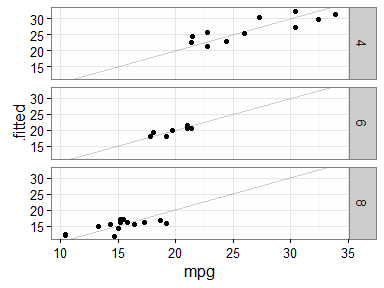This figure is showing us the fitted results of three separate regression analyses: one for each subset of the `mtcars` data corresponding to cars with 4, 6, or 8 cylinders. As we know from above, the R2 value for cars with 8 cylinders is lowest, and it’s somewhat evident from this plot (though the small sample sizes make it difficult to feel confident).

## randomForest example #

For anyone looking to sink their teeth into something a little more complex, below is a fully worked example of examining the relative importance of variables in a `randomForest()` model. The model predicts the arrival delay of flights using time-related variables (departure time, year, month and day). Relevant to this post, we fit this model to the data separately for each of three airline carriers.

Notice that this implements the same code we’ve been using so far, with just a few tweaks to select an appropriate data set and obtain information from the fitted models.

The resulting plot suggests to us that the importance of a flight’s `day` for predicting it’s arrival delay varies depending on the carrier. Specifically, it is reasonably informative for predicting the arrival delay of Pinnacle Airlines (`9E`), not so useful for Virgin America (`VX`), and practically useless for Alaska Airlines (`AS`).

``````library(randomForest)
library(nycflights13)

# Convenience function to get importance information from a randomForest fit
# into a data frame
imp_df <- function(rf_fit) {
imp <- randomForest::importance(rf_fit)
vars <- rownames(imp)
imp %>%
tibble::as_tibble() %>%
dplyr::mutate(var = vars)
}

set.seed(123)
flights %>%
# Selecting data to work with
na.omit() %>%
select(carrier, arr_delay, year, month, day, dep_time) %>%
filter(carrier %in% c("9E", "AS", "VX")) %>%
# Nesting data and fitting model
nest(-carrier) %>%
mutate(fit = map(data, ~ randomForest(arr_delay ~ ., data = .,
importance = TRUE,
ntree = 100)),
importance = map(fit, imp_df)) %>%
# Unnesting and plotting
unnest(importance) %>%
ggplot(aes(x = `%IncMSE`, y = var, color = `%IncMSE`)) +
geom_segment(aes(xend = min(`%IncMSE`), yend = var), alpha = .2) +
geom_point(size = 3) +
facet_grid(. ~ carrier) +
guides(color = "none") +
theme_bw()
``````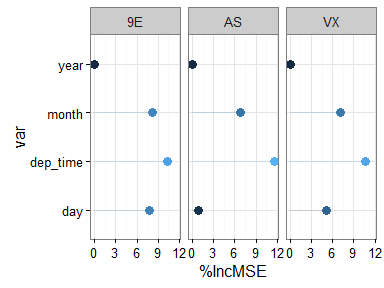## Sign off #

Thanks for reading and I hope this was useful for you.

For updates of recent blog posts, follow @drsimonj on Twitter, or email me at drsimonjackson@gmail.com to get in touch.

If you’d like the code that produced this blog, check out the blogR GitHub repository.